The LP Procedure
 An Introductory Example

A simple blending problem illustrates the dense and sparse input formats and the use of PROC LP. A step in refining crude oil into finished oil products involves a distillation process that splits crude into various streams. Suppose there are three types of crude available: Arabian light, Arabian heavy, and Brega. These types of crude are distilled into light naphtha, intermediate naphtha, and heating oil. These in turn are blended into jet fuel using one of two recipes. What amounts of the three crudes maximize the profit from producing jet fuel? A formulation to answer this question is as follows: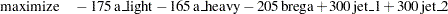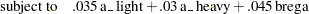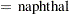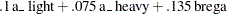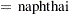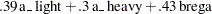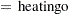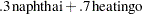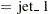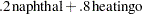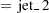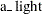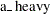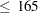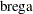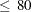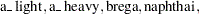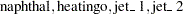The following data set gives the representation of this formulation. Notice that the variable names are the structural variables, the rows are the constraints, and the coefficients are given as the values for the structural variables.

```   data;
input _id_ \$17.
a_light a_heavy brega naphthal naphthai
heatingo jet_1 jet_2
_type_ \$ _rhs_;
datalines;
profit            -175 -165 -205  0  0  0 300 300 max     .
naphtha_l_conv    .035 .030 .045 -1  0  0   0   0  eq     0
naphtha_i_conv    .100 .075 .135  0 -1  0   0   0  eq     0
heating_o_conv    .390 .300 .430  0  0 -1   0   0  eq     0
recipe_1             0    0    0  0 .3 .7  -1   0  eq     0
recipe_2             0    0    0 .2  0 .8   0  -1  eq     0
available          110  165   80  .  .  .   .   . upperbd .
;
```

The same model can be specified in the sparse format, as follows. This format enables you to omit the zero coefficients.

```   data;
format _type_ \$8. _col_ \$8. _row_ \$16. ;
input _type_ \$ _col_ \$ _row_ \$ _coef_ ;
datalines;
max      .             profit                    .
eq       .             napha_l_conv              .
eq       .             napha_i_conv              .
eq       .             heating_oil_conv          .
eq       .             recipe_1                  .
eq       .             recipe_2                  .
upperbd  .             available                 .
.        a_light       profit                 -175
.        a_light       napha_l_conv           .035
.        a_light       napha_i_conv           .100
.        a_light       heating_oil_conv       .390
.        a_light       available               110
.        a_heavy       profit                 -165
.        a_heavy       napha_l_conv           .030
.        a_heavy       napha_i_conv           .075
.        a_heavy       heating_oil_conv       .300
.        a_heavy       available               165
.        brega         profit                 -205
.        brega         napha_l_conv           .045
.        brega         napha_i_conv           .135
.        brega         heating_oil_conv       .430
.        brega         available                80
.        naphthal      napha_l_conv             -1
.        naphthal      recipe_2                 .2
.        naphthai      napha_i_conv             -1
.        naphthai      recipe_1                 .3
.        heatingo      heating_oil_conv         -1
.        heatingo      recipe_1                 .7
.        heatingo      recipe_2                 .8
.        jet_1         profit                  300
.        jet_1         recipe_1                 -1
.        jet_2         profit                  300
.        jet_2         recipe_2                 -1
.        _rhs_         recipe_1                  0
;
```

Because the input order of the model into PROC LP is unimportant, this model can be specified in sparse input in arbitrary row order. Example 5.2 in the section Examples: LP Procedure demonstrates this.

The dense and sparse forms of model input give you flexibility to generate models using the SAS language. The dense form of the model is solved with the statements

```   proc lp;
run;
```

The sparse form is solved with the statements

```   proc lp sparsedata;
run;
```

Example 5.1 and Example 5.2 in the section Examples: LP Procedure continue with this problem.

### Problem Input

As default, PROC LP uses the most recently created SAS data set as the problem input data set. However, if you want to input the problem from a specific SAS data set, use the DATA= option. For example, if the previous dense form data set has the name DENSE, the PROC LP statements can be written as

```   proc lp data=dense;
run;
```

### Problem Definition Statements

In the previous dense form data set, the _ID_, _TYPE_, and _RHS_ variables are special variables in PROC LP. They stand for id variable, type variable, and right-hand-side variable. If you replace those variable names with, for example, ROWNAME, TYPE, and RHS, you need the problem definition statements (ID, TYPE and RHS) in PROC LP:

```   proc lp;
id rowname;
type type;
rhs rhs;
run;
```

Other special variables for the dense format are _RHSSEN_ and _RANGE_, which identify the vectors for the right-hand-side sensitivity and range analyses. The corresponding statements are the RHSSEN and RANGE statements. (Notice that a variable name can be identical to a statement name.)

In the same way, if you replace the variables _COL_, _ROW_, _TYPE_, and _COEF_ in the previous sparse form data set by COLUMN, ROW, TYPE, and COEF, you need the problem definition statements (COL, ROW, TYPE, and COEF) in PROC LP.

```   proc lp sparsedata;
col column;
row row;
type type;
coef coef;
run;
```

In the sparse form data set, the value '_RHS_' under the variable _COL_ is a special column name, which represents the model’s right-hand-side column. If you replace it by a value 'R', the PROC LP statements would be

```   proc lp sparsedata;
rhs r;
run;
```

Other special column names for the sparse format are '_RHSSEN_' and '_RANGE_'. The corresponding statements are the RHSSEN and RANGE statements.

PROC LP is case insensitive to variable names and all character values, including the row and column names in the sparse format. The order of the problem definition statements is not important.

For the dense format, a model’s row names appear as character values in a SAS data set. For the sparse format, both the row and the column names of the model appear as character values in the data set. Thus, you can put spaces or other special characters in the names. When referring to these names in the problem definition statement or other LP statements, you must use single or double quotes around them. For example, if you replace '_RHS_' by 'R H S' in the previous sparse form data set, the PROC LP statements would become

```   proc lp sparsedata;
rhs "r h s";
run;
```

### LP Options

The specifications SPARSEDATA and DATA= in the previous examples are PROC LP options. PROC LP options include

### Interactive Processing

Interactive control options include READPAUSE, ENDPAUSE, and so forth. You can run PROC LP interactively using those options. For example, for the blending problem example in the dense form, you can first pause the procedure before iterations start with the READPAUSE option. The PROC LP statements are

```   proc lp readpause;
run;
```

When the procedure pauses, you run the PRINT statement to display the initial technological matrix and see if the input is correct. Then you run the PIVOT statement to do one simplex pivot and pause. After that you use the SHOW statement to check the current solution status. Then you apply the RESET statement to tell the procedure to stop as soon as it finds a solution. Now you use the RUN statement to continue the execution. When the procedure stops, you run the PRINT statement again to do a price range analysis and QUIT the procedure. Use a SAS %PUT statement to display the contents of PROC LP’s macro variable, _ORLP_, which contains iterations and solution information. What follows are the complete statements in batch mode:

```   proc lp readpause;
run;
print matrix(,); /* display all rows and columns. */
pivot;
show status;
reset endpause;
run;
print rangeprice;
quit;
%put &_orlp_;
```

Note:You can force PROC LP to pause during iterations by using the CTRL-BREAK key.Previous Page | Next Page | Top of Page